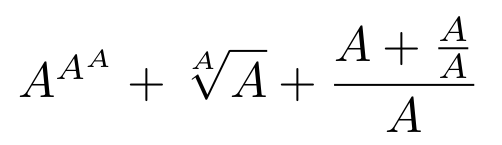# math-script-level and math-style explainer

This document proposes two new CSS properties math-style and math-script-level controlling how the font-size evolves inside mathematical constructions. More generally math-style may be used to indicate to implementations of math layout whether logical height should be minimized. For further discussions on this proposal see math-script-level and math-style comments.

## Examples

• <div class="my-underover"><div>Base</div><div>Overscript</div><div>Underscript</div></div> and .my-underover > :not(:first-child) { math-script-level: add(1); } would scale down the font size in under and over scripts.

• In the previous example, one could do <div style="math-script-level: 0;">Overscript</div> to reset the font-size in the overscript back to the initial base size.

• <div class="my-fraction"><div>Numerator</div><div>Denominator</div></div> and .my-fraction > * { math-script-level: auto; } would scale down the font size in the numerator or denominator, depending on the actual math-style on the the fraction.

• The LaTeX formula $$A^{A^A} + \sqrt[A]{A} + \frac{A+\frac{A}{A}}{A}$$ uses text of different sizes. One could style equivalent HTML nodes with the font-size property but it could be more convenient to set the math-script-level (base, scripts, numerator/denominator) and math-style values (initially ‘display’ mode but ‘inline’ mode in inner constructions).• Polyfills and native implementations can emulate MathML behavior such as <mstyle displaystyle="true">...</mstyle>, <mstyle scriptlevel="2">...</mstyle> or <mstyle scriptlevel="+3">...</mstyle> by mapping to math-style: display, math-script-level: 2; and math-script-level: add(3); respectively.

## Rationale

• Mathematical formulas are made of several nested constructions. Readers expect that each time you go deeper in the expressions (e.g. enter a superscript) text will be automatically scaled down. This is can be achieved by adding relative or absolute font-size rules on each relevant node, but that’s a bit tedious and error-prone.

• The actual rules for the text size change are a bit complex, and involve concepts similar to math-script-level (how deep we are in the expression) and math-style (whether we try to minimize logical height). In the TeX example $$A^{A^A} + \sqrt[A]{A} + \frac{A+\frac{A}{A}}{A}$$, the A letter is scaled down when entering the superscripts but even faster when entering a root’s prescript. Also it is scaled down when entering the inner fraction but not when entering the outer one. These cases on a basic example suggest that a standardized approach would be important to ensure interoperability.

• More generally, mathematical typesetting follows rules to decide whether math layout should try and minimize the logical height and this decision can change when going deeper in the expressions or by user request. In the previous example this affects whether we increase the math-script-level when entering fractions but there are more of them such as changing the layout of under/over scripts, reducing spacing or fraction’s line thickness, etc Again, it’s handy to use CSS for the inheritance and overriding of this math-style value even if most of its effect happens in the layout and painting phase.

• math-script-level and math-style are purely stylistic features so it makes sense to have them integrated in the style system.

• These properties intend to implement the following MathML attributes: display, displaystyle and scriptlevel attributes. Again, there are complex interactions between these attributes, MathML elements and font-size. Hence relying on native CSS properties seems the proper way to implement it.

• These properties can be used to emulate TeX’s \displaystyle, \textstyle, \scriptstyle, and \scriptscriptstyle modes, they correspond to math-style and math-script-level as “display” and 0, “inline” and 0, “inline” and 1, and inline and 2, respectively. These are important use cases requested by math people.

• OpenType math fonts have values about how the script should scale down when going from script level 0 to script level 1 (scriptPercentScaleDown) or 2 (scriptScriptPercentScaleDown).

## Status in Web Engines

• The displaystyle and scriptlevel attributes are implemented in Gecko and Stylo via an internal CSS property. Actually, more features are implemented and maybe this is an opportunity to simplify things.
• The displaystyle attribute is implemented in WebKit using some custom CSS-like inheritance. It could be rewritten to rely on math-style instead. The default scriptlevel behavior is implemented but does take into account user-specified attribute and does not interact with displaystyle. Relying on math-script-level would allow a cleaner and more complete implementation.
• displaystyle and scriptlevel attributes are tested in MathML WPT, Gecko and WebKit tests. Having these separate properties could allow to do pure CSS tests related to the interaction with font-size.

## Proposal

### CSS math-style property

See https://mathml-refresh.github.io/mathml-core/#the-math-style-property

### CSS math-script-level property

See https://mathml-refresh.github.io/mathml-core/#the-math-script-level-property

### Native implementations of display, displaystyle and scriptlevel

See https://mathml-refresh.github.io/mathml-core/#attribute-display, https://mathml-refresh.github.io/mathml-core/#attribute-displaystyle and https://mathml-refresh.github.io/mathml-core/#attribute-scriptlevel